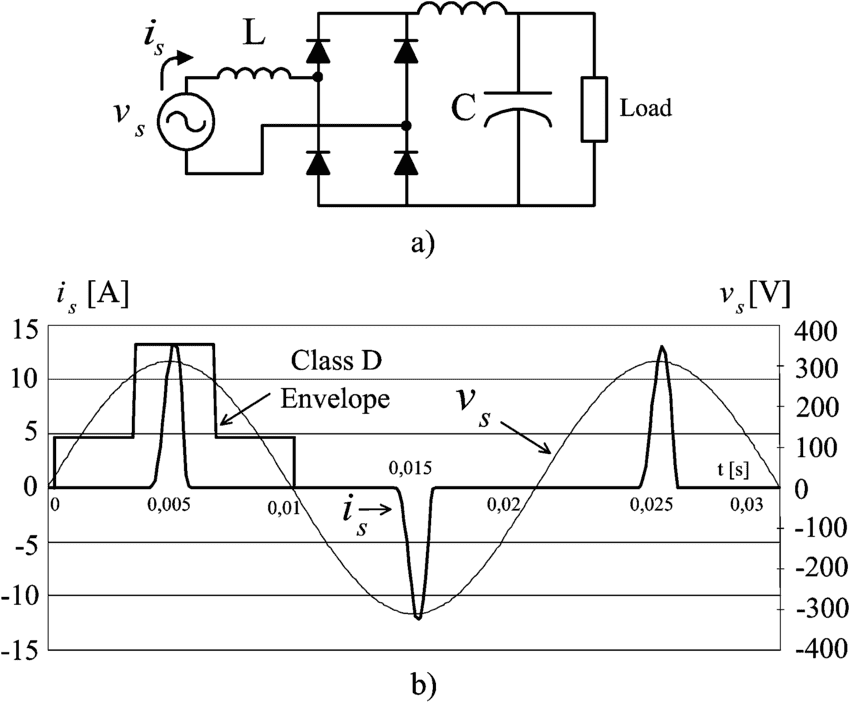# [Equation Validity] - Current Characterization (AC) in Sockets

HAgdn
Homework Statement:
I am trying mathematically characterize the current running a wire connected to the AC mains. This wire has a socket and can take n number of loads linear/non-linear load.

I assumed this:

Considering that the loads have different current draws and the possibility of non-linear load. And the current running through the wire is the sum of the draw current of the loads (is this valid to say?). Since the overall current running through the wire is the sum of all the draw current of the loads (which is periodic), then, Fourier's theorem can be applied by which the theorem states that a wave is composed of sinusoidal components having a proper amplitude and frequency. And such wave is the "REAL" current running through the wire when loads are connected.
Relevant Equations:
Through my assumption, what I got is:

I = A_0 * sin(n_0wt + p) + A_1 * sin(n_1wt+p) + ... +A_n * sin(n_nwt+p)

Where:

I = current running through the wire connected to the AC mains whose socket whose socket has loads connected.

A_n = the draw current of an nth load

w = angular frequency

p = phase shift
I = A_0 * sin(n_0wt + p) + A_1 * sin(n_1wt + p) + ... +A_n * sin(n_nwt + p)

Looking at the equation, it only contains sinusoidal waves. Further, there is the possibility of waves having the same shift or no shift at all and even, having the same frequency. Is it really valid or correct to use the concept of Fourier in this scenario? I am trying to use the equation in a study of mine.

Homework Helper
$$I = A_0 * sin(n_0\omega t + p) + A_1 * sin(n_1\omega t + p) + ... +A_n * sin(n_n\omega t + p)$$
Looking at the equation, it only contains sinusoidal waves. Further, there is the possibility of waves having the same shift or no shift at all and even, having the same frequency
When I look, I see
• Only a single ##p##, so all loads show the same phase lag ?
• multiple frequencies, which is strange: the mains only has one frequency
So: no, I don't think you are on the right path. Advice: read up on AC circuit analysis.

Mentor
multiple frequencies, which is strange: the mains only has one frequency
The AC Mains voltage does have some low-order distortions and noise, but even assuming a perfect sinusoidal voltage, the current I(t) will depend on the load. If the load is non-linear, that can generate harmonic content in the I(t) waveform.

A good example is the current drawn by the common full-wave rectifier input stage for many power supplies:

https://www.researchgate.net/profile/Pablo_Lezana_Illesca/publication/3218408/figure/fig7/AS:668537572057093@1536403186524/Single-phase-rectifier-a-Circuit-b-Waveforms-of-the-input-voltage-and-current.pngHomework Helper
Gold Member
2022 Award
multiple frequencies, which is strange: the mains only has one frequency
Since the loads are nonlinear the current will not be a pure sine wave. According to M. Fourier that means there will be harmonics.

•